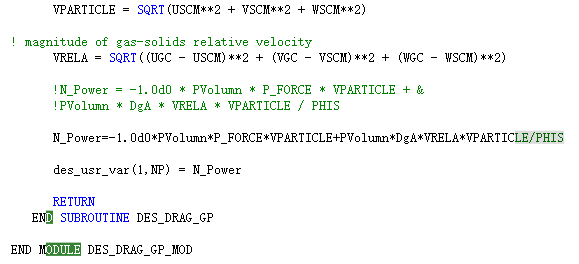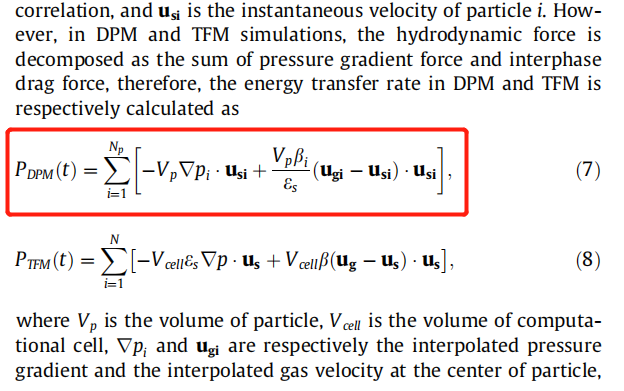# Error: Incompatible ranks 0 and 2 in assignment at (1)

Hi, here is my code, which is added in drag_gp_des.f.In the formula for calculating N_Power, all variables are of DOUBLE PRECISION type.

When I build the solver, an error is reported when I build the solver.

How can I resolve this error?

Best regards
xjwuyuhao

The formula for calculating N_Power corresponds to formula (7) in the figure below:Any suggestion would be appreciated.